# Analyzing the Gas Phase Spectrum of Hydrogen Chloride with FT-IR

Infrared spectroscopy is a standard technique, which depends upon the interaction between infrared radiation and the vibrating dipole moments of molecules. Except for homonuclear diatomics and noble gases, a characteristic spectrum for each substance is generated in the vapor phase. This individual spectrum is derived from the exclusive physical properties of each molecule that is studied. Infrared spectroscopy serves as a remarkable tool for obtaining the values of these physical properties, especially the molecular structure and force constants of the chemical bonds. An ideal example to demonstrate the principles is the phase spectrum of the heteronuclear diatomic molecule hydrogen chloride (HCl).

When measuring the infrared radiation absorbed by a liquid or solid, well-defined regions of absorption can be attributed to the vibrational transitions. Assuming that the resolution of the instrument is sufficient, the IR spectra of the gas phase molecules do not exhibit such smooth and structureless absorption bands but rather absorption regions of significant complexity. The fine structure of these absorption bands can be correlated to changes in the molecule’s rotational energy accompanied by a vibrational transition.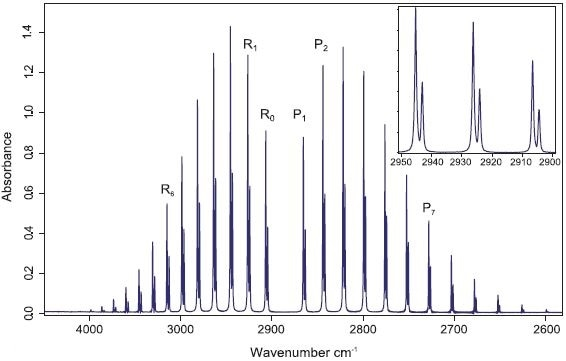FT-IR spectrum of hydrogen chloride in the gas phase. Insert: The ro-vibrational lines are split due to the chlorine 35 and chlorine 37 natural isotopic ratio.

## Simple Theory

In order to describe molecular motions, one begins with simple physical models in which the atoms are considered to be points of mass held together by weightless elastic springs. The diatomic one, which has two mass points connected by a spring, is the simplest molecule to be built this way. Classical mechanics gives the corresponding vibrational equation of this molecule; the solution is represented by a sinusoidal motion of two masses at a frequency determined by their masses and the restoring force of the spring. Although the potential and the kinetic energy of the system continually interconvert during the vibration, the sum of both energies keeps constant as in a pendulum.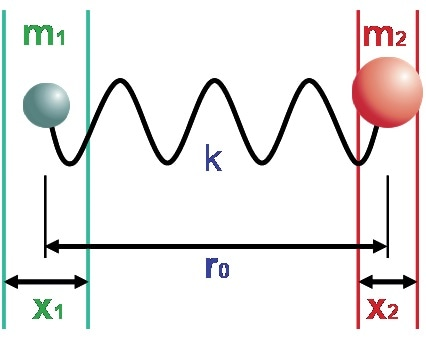Figure 1. Mechanical model of the vibrating diatomic molecule. k the spring constant corresponds to the molecular binding force, m1 and m2 are the atomic mass and r0 the equilibrium distance.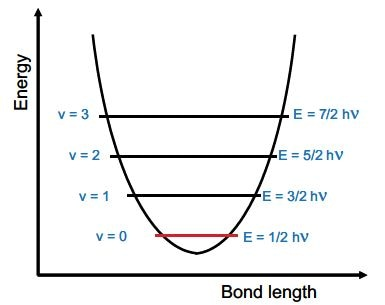Figure 2. The potential energy of the two-mass model can be assumed to be a parabola, that is harmonic. The further the masses are displaced from their equilibrium position, the higher the potential energy of the system.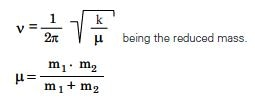The resulting classical vibrational frequency of such an oscillator is given by:

The quantum mechanical description of the harmonic oscillator can be used to describe the vibrational motion of a heteronuclear diatomic molecule. The quantized energy levels are given by:

Ev = hν (v+1/2)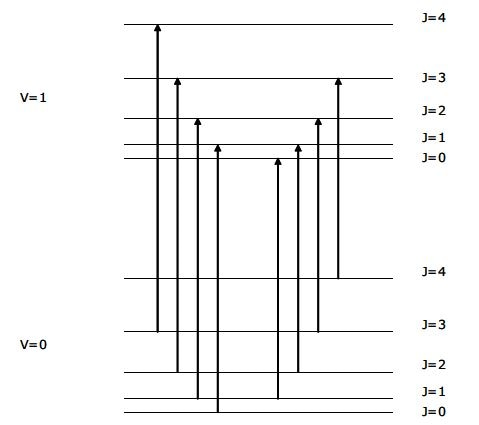Vibrational and rotational energy levels for a diatomic molecule such as HCl. The spacing between V=0 and V=1 is much more than depicted. The R branch ro-vibrational lines are shown on the left and the P branch ro-vibrational lines are shown on the right. Only the first few J levels are shown.

Where h is Planck’s constant, ν is the classical vibrational frequency, and Ev is the energy of the vibrational state with quantum number v. v can take any integer value starting from 0.

Under regular conditions, the vibrational transitions occur from the ground state (v=0) to the first excited state (v=1). This simple vibrational model predicts just one single vibrational band for a diatomic molecule. However, a series of closely spaced bands as for the spectrum of HCl can be observed in the figure. These lines are because of the rotational sub-structure, and a further quantum number J for the rotational energy is introduced. J also takes integer values starting from 0. Therefore, every vibrational level has a stack of rotational levels and transitions take place from v=0 to v=1 with J changing by –1 (so called P branch lines) and J changing by +1 (so called R branch lines).

A few examples where lines are assigned and the number in brackets refers to the J number of the v=0 level are shown in the figure for the HCl spectrum. It turns out that the Q branch line with no change in J and expected at the band center is missing because of the operation of selection rules. This leads to a gap between R(0) and P(1), see the figure.

The rotational energies are given by BJ(J+1), where B is called the rotational constant. Knowledge of B leads to the molecular structure determination or, in this simple case, the bond length. To a first approximation, the rotational constants B of the v=1 (upper) and v=0 (lower) vibrational levels are determined as follows. For every J, the differences in cm-1 positions of the pairs of lines shown on the left side of the two equations are taken and divided by (2J+1).

R(J-1) – P(J+1) = 2Blower (2J+1)
R(J) – P(J) = 2Bupper (2J+1)

J R(J) P(J) R(J-1)-P(J+1) R(J)-P(J) 2J+1 2Blower 2Bupper (2J+1)**2
0 2906.25
1 2925.9 2865.09 62.63 60.81 3 20.877 20.27 9
2 2944.92 2843.62 104.35 101.3 5 20.87 20.26 25
3 2963.29 2821.55 146 141.74 7 20.857 20.249 49
4 2981.01 2798.92 187.55 182.09 9 20.839 20.232 81
5 2998.05 2775.74 229 222.31 11 20.818 20.21 121
6 3014.42 2752.01 270.29 262.41 13 20.792 20.185 169
7 3030.1 2727.76 311.44 302.34 15 20.763 20.156 225
8 3045.07 2702.98 352.39 342.09 17 20.729 20.123 289
9 3059.32 2677.71 393.13 381.61 19 20.691 20.085 361
10 3072.85 2651.94 433.6 420.91 21 20.648 20.043 441
11 3085.66 2625.72 473.86 459.94 23 20.603 19.997 529
12   2598.99

J R(J) P(J) R(J-1)-P(J+1) R(J)-P(J) 2J+1 2Blower 2Bupper (2J+1)**2
0 2904.08
1 2923.71 2862.99 62.52 60.72 3 20.84 20.24 9
2 2942.7 2841.56 104.17 101.14 5 20.834 20.228 25
3 2961.05 2819.54 145.75 141.51 7 20.821 20.216 49
4 2978.74 2796.95 187.24 181.79 9 20.804 20.199 81
5 2995.76 2773.81 228.62 221.95 11 20.784 20.177 121
6 3012.11 2750.12 269.84 261.99 13 20.757 20.153 169
7 3027.76 2725.92 310.9 301.84 15 20.727 20.123 225
8 3042.72 2701.21 351.76 341.51 17 20.692 20.089 289
9 3056.92 2676 392.44 380.92 19 20.655 20.048 361
10 3070.51 2650.28 432.79 420.23 21 20.609 20.011 441
11   2624.13     23     529

B = Rotational constant; Delta J = +1 is the R branch, Delta J = -1 is the P branch

This can be carried out for both chlorine isotopes (Cl-35 and Cl- 37), see next two tables.

## Extended Theory

Examination of the B constants determined in this simple way displays a distinct curvature with J number. This emerges from the neglect of the centrifugal distortion of the HCl molecule, which increases with J rotational quantum number. As the molecule stretches at higher rotational energy, its moment of inertia I increases. B and the moment of inertia are inversely related to, and therefore B decreases with J. Moreover, B has a slightly lower value in the upper (v=1) vibrational state.

Note: I = μr2 where μ = reduced mass and r = internuclear distance

μ = MHMCl/(MH + MCL) where M = atomic masses

More detailed theory leads to the revised equations:

R(J-1) – P(J+1) = (2Blower – 3Dlower)(2J+1) – Dlower (2J+1)3
R(J) – P(J) = (2Bupper – 3Dupper)(2J+1) – Dupper(2J+1)3

Where D = centrifugal distortion constant, which has a small value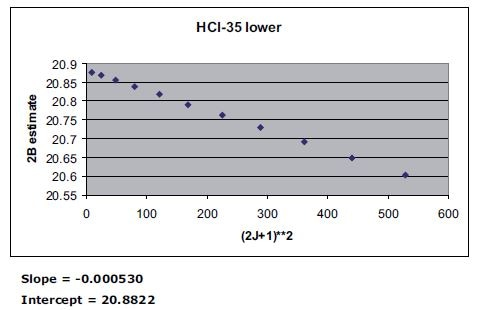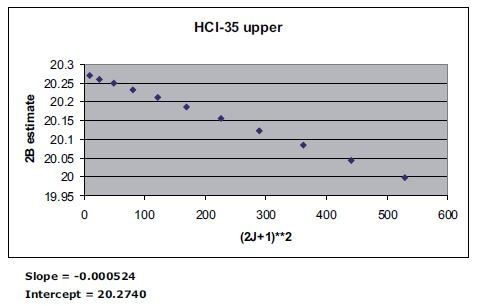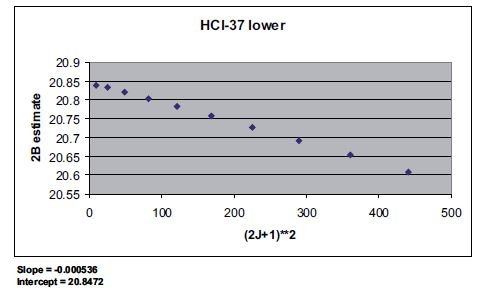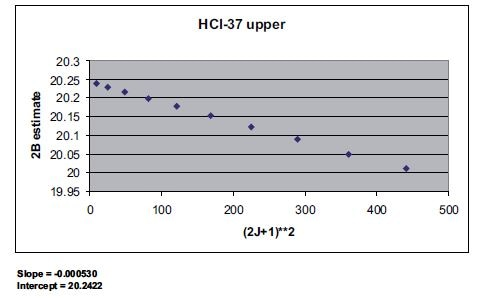A plot of the previously determined 2B values versus (2J+1)2 will result in a straight line. The intercept will be (2B-3D) and the slope – D.

## Calculation of the Internuclear Distance

The rotational constants should be extrapolated to the equilibrium state when the molecule would reside hypothetically at the bottom of the potential well.

HCL-35 Literature HCL-37
B lower 10.442 10.440254 10.424
B upper 10.138 10.136228 10.122
D lower 0.00053 0.00052828 0.000536
D upper 0.000524 0.00052157 0.00053

Values in cm-1
Literature values for HCl-35 taken from very high-resolution spectroscopy – Journal of Molecular Spectroscopy, vol. 17, 1965, p. 122.

Blower = Bequilibrium – 0.5 α
Bupper = Bequilibrium – 1.5 α

HCL-35 HCL-37
B equilibrium 10.594 10.576
alpha 0.304 0.302

Values in cm-1

Where α = vibration – rotation interaction constant (α = alpha in the table)

I = 16.8575/B gives the moment of inertia I, where B is in units of cm-1 and I has units of amu Angstroms2 (1 Angstrom = 10-8 cm). The number in this equation derives from the quantity h/8π2c where c = velocity of light.

The literature value for the HCl equilibrium bond length determined by microwave spectroscopy is 1.27455 Angstroms (Phys. Rev., vol 136, A1229, (1964)). From a theoretical standpoint, these bond length values for HCl-35 and HCl-37 should be the same.

This experiment with the ALPHA FT-IR spectrometer shows excellent agreement with these facts.

HCL-35 HCL-37
B equilibrium 10.594 10.576
I (amu Angstroms**2) 1.5912 1.594
Reduced mass (amu) 0.979569 0.981054
Internuclear distance (Angstroms) 1.2745 1.2747

## Derivation of the Vibrational Band Origin V0-1

 V0-1 = R(0) – (2Bupper – 4Dupper) Equation 1
 V0-1 = P(1) + (2Blower – 4Dlower) Equation 2

The effect of D can be neglected.

Assuming a harmonic oscillator, the band origins for HCl-37 and HCl-35 are related by:

V0-1 (HCl-37) = V0-1 (HCl-35) * √ (reduced mass HCl-35/reduced mass HCl-37)

Using values of the reduced mass for HCl-35 and HCl-37 from the table, the prediction for HCl-37 is 2883.78 cm-1 when compared to the experimental value of 2883.84 cm-1.

HCL-35 HCL-37
eqn 1 2885.97 2883.84
eqn 2 2885.97 2883.84

Values in cm-1, literature value for HCl-35 is 2885.9775 cm-1, J. Mol. Spectroscopy vol. 17, 1965, p.122

## Conclusions

The ALPHA II is an economical and compact FT-IR spectrometer that is ideally suited for both teaching applications and QA/QC. With the high-resolution option for the ALPHA II FT-IR spectrometer, one can obtain excellent HCl vapor spectra measured in a gas cell. Analysis of the HCl spectrum, making full use of the OPUS peak pick software installed on the ALPHA II Touch-PC or Windows PC, gives molecular constants that are fully consistent with the values published in the spectroscopic research literature.

These outstanding experimental results are possible, thanks to the excellent signal to noise ratio, good line shape, and precise frequency registration of the ALPHA II FT-IR spectrometer.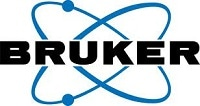This information has been sourced, reviewed and adapted from materials provided by Bruker Optics.

## Citations

• APA

Bruker Optics. (2019, August 28). Analyzing the Gas Phase Spectrum of Hydrogen Chloride with FT-IR. AZoM. Retrieved on October 28, 2020 from https://www.azom.com/article.aspx?ArticleID=15226.

• MLA

Bruker Optics. "Analyzing the Gas Phase Spectrum of Hydrogen Chloride with FT-IR". AZoM. 28 October 2020. <https://www.azom.com/article.aspx?ArticleID=15226>.

• Chicago

Bruker Optics. "Analyzing the Gas Phase Spectrum of Hydrogen Chloride with FT-IR". AZoM. https://www.azom.com/article.aspx?ArticleID=15226. (accessed October 28, 2020).

• Harvard

Bruker Optics. 2019. Analyzing the Gas Phase Spectrum of Hydrogen Chloride with FT-IR. AZoM, viewed 28 October 2020, https://www.azom.com/article.aspx?ArticleID=15226.